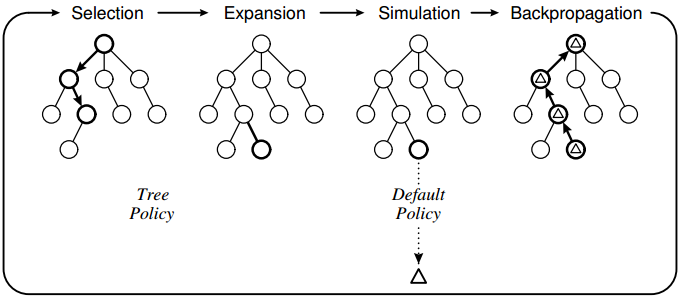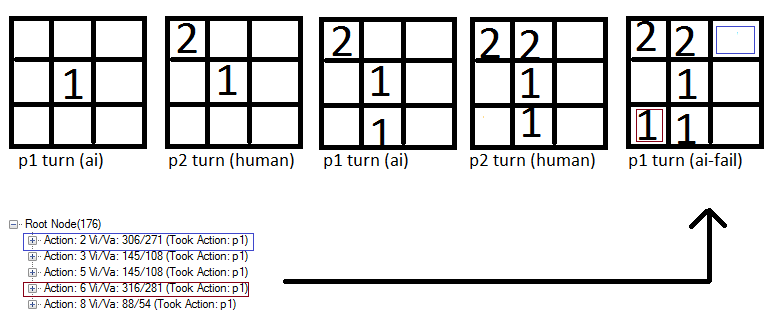2 views

To learn about MCTS (Monte Carlo Tree Search) I've used the algorithm to make an AI for the classic game of tic-tac-toe. I have implemented the algorithm using the following design:The tree policy is based on UCT and the default policy is to perform random moves until the game ends. What I have observed with my implementation is that the computer sometimes makes erroneous moves because it fails to "see" that a particular move will result in a loss directly.

For instance:Notice how the action 6 (red square) is valued slightly higher than the blue square and therefore the computer marks this spot. I think this is because the game policy is based on random moves and therefore a good chance exists that the human will not put a "2" in the blue box. And if the player does not put a 2 in the blue box, the computer is guaranteed a win.

My Questions

1) Is this a known issue with MCTS or is it a result of a failed implementation?

2) What could be possible solutions? I'm thinking about confining the moves in the selection phase but I'm not sure :-)

The code for the core MCTS:

BestChildUCB(Node current, double C) { Node bestChild = null; double best = double.NegativeInfinity; foreach (Node child in current.children) { double UCB1 = ((double)child.value / (double)child.visits) + C * Math.Sqrt((2.0 * Math.Log((double)current.visits)) / (double)child.visits); if (UCB1 > best) { bestChild = child; best = UCB1; } } return bestChild; } //#2. Expand a node by creating a new move and returning the node public Node Expand(Node current, Game game) { //Copy current state to the game helper.CopyBytes(game.board, current.state); List<byte> validMoves = game.GetValidMoves(current.state); for (int i = 0; i < validMoves.Count; i++) { //We already have evaluated this move if (current.children.Exists(a => a.action == validMoves[i])) continue; int playerActing = Opponent(current.PlayerTookAction); Node node = new Node(current, validMoves[i], playerActing); current.children.Add(node); //Do the move in the game and save it to the child node game.Mark(playerActing, validMoves[i]); helper.CopyBytes(node.state, game.board); //Return to the previous game state helper.CopyBytes(game.board, current.state); return node; } throw new Exception("Error"); } //#3. Roll-out. Simulate a game with a given policy and return the value public int Rollout(Node current, Game game, int startPlayer) { Random r = new Random(1337); helper.CopyBytes(game.board, current.state); int player = Opponent(current.PlayerTookAction); //Do the policy until a winner is found for the first (change?) node added while (game.GetWinner() == 0) { //Random List<byte> moves = game.GetValidMoves(); byte move = moves[r.Next(0, moves.Count)]; game.Mark(player, move); player = Opponent(player); } if (game.GetWinner() == startPlayer) return 1; return 0; } //#4. Update public unsafe void Update(Node current, int value) { do { current.visits++; current.value += value; current = current.parent; } while (current != null); }

by (108k points)
edited by

Answering your first question, If MCTS is used in its basic form without any improvements, it may fail to suggest reasonable moves. It may happen if nodes are not visited adequately which results in inaccurate estimates.

However, MCTS can be improved using some techniques. It involves domain specific as well as domain-independent techniques.

In domain-specific techniques, the simulation stage produces more realistic play outs rather than stochastic simulations. Though it requires knowledge of game-specific techniques and rules.

Now coming to your second question: I think the problem was that the search space was too small. This ensures that even if the selection does select a move that is actually terminal, this move is never chosen and resources are used to explore other moves instead. You can add the following code in your program:

//If this move is terminal and the opponent wins, this means we have previously made a move where the opponent can always find a move to win

if (game.GetWinner() == Opponent(startPlayer))

current.parent.value = int.MinValue;

return 0;

}

If you want to make your career in Artificial Intelligence then go through this video:

For the best of career growth, check out Artificial Intelligence Online Course and get certified.